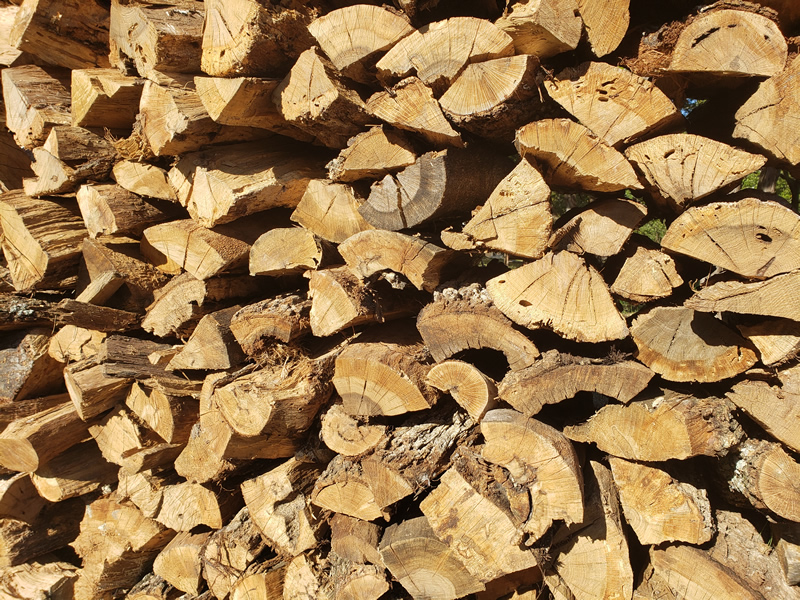Texas Butcher Paper & Firewood

## Cord of Firewood – A Standard Unit of Measurement

• Post author:
• Post category:News

1/4 Cord is 4 foot high 4 foot long, and 16-18 inches wide

But using the following Firewood & Cord Calculations Calculator, can determine more info.
Note: must input 24 inches for width
32.00 cubic feet
0.90 cubic metres
0.25 cords

A cord of firewood measures 4′ x 4′ x 8′ and contains 128 cubic feet. You can calculate the size of a stack in cubic feet by measuring the height, depth, and length of the stack and multiplying one by the other two. For instance:

4′ high x 1.5′ deep x 48′ long = 288 cubic feet

To figure out how many cord is in that same stack, simple divide it’s size in cubic feet by the 128 cubic feet that are in a cord.

288 cubic feet divided by 128 cubic feet = 2.25 cord

A cord of firewood measures 4′ x 4′ x 8′ and contains 128 cubic feet. You can calculate the size of a stack in cubic feet by measuring the height, depth, and length of the stack and multiplying one by the other two. For instance:

4′ high x 1.5′ deep x 48′ long = 288 cubic feet

64.00 cubic feet
1.81 cubic metres
0.50 cords

A cord of firewood measures 4′ x 4′ x 8′ and contains 128 cubic feet. You can calculate the size of a stack in cubic feet by measuring the height, depth, and length of the stack and multiplying one by the other two. For instance:

4′ high x 1.5′ deep x 48′ long = 288 cubic feet

96.00 cubic feet
2.71 cubic metres
0.75 cords

128.00 cubic feet
3.62 cubic metres
1 cord